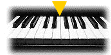Elementary Algebra Assignment #32A1. Determine which of the ordered pairs are solutions for the equation 3x - 2y = 12 (0, 6), (0, -6), (4, 0), (-2, 0) 2. Determine which of the ordered pairs are solutions for the equation 7x - y = 7 (0, 7), (0, -7), (4, 0), (1, 0) 3. Determine which of the ordered pairs are solutions for the equation 5x - 3y = 13 (0, -13/3), (0, 13/3), (3, 2/3), (-2, 0) 4. Complete the ordered pair so that each is a solution for the equation: 4x - y = 8 (0, ), ( , 0), (1, ), ( ,-4) 5. Complete the ordered pairs so that each is a solution to the equation x - 2y = 8 (0, ), (, 0), (1, ), ( , -2) 6. Complete the ordered pairs so that each is a solution to the equation x - y = 6 (0, ), ( , 0 ), ( ,-3), (-1, )BACK TO ASSIGNMENTS